## Wavelet Families

The Wavelet Toolbox™ software includes a large number of wavelets that you can use for both continuous and discrete analysis. For discrete analysis, examples include orthogonal wavelets (Daubechies’ extremal phase and least asymmetric wavelets) and B-spline biorthogonal wavelets. For continuous analysis, the Wavelet Toolbox software includes Morlet, Meyer, derivative of Gaussian, and Paul wavelets.

The choice of wavelet is dictated by the signal or image characteristics and the nature of the application. If you understand the properties of the analysis and synthesis wavelet, you can choose a wavelet that is optimized for your application.

Wavelet families vary in terms of several important properties. Examples include:

• Support of the wavelet in time and frequency and rate of decay.

• Symmetry or antisymmetry of the wavelet. The accompanying perfect reconstruction filters have linear phase.

• Number of vanishing moments. Wavelets with increasing numbers of vanishing moments result in sparse representations for a large class of signals and images.

• Regularity of the wavelet. Smoother wavelets provide sharper frequency resolution. Additionally, iterative algorithms for wavelet construction converge faster.

• Existence of a scaling function, φ.

For continuous analysis, the Wavelet Toolbox software analytic wavelet-based analysis for select wavelets. See `cwt` and `icwt` for details. Inverse Continuous Wavelet Transform for a basic theoretical motivation. CWT-Based Time-Frequency Analysis illustrates the use of the continuous wavelet transform for simulated and real-world signals.

Entering `waveinfo` at the command line displays a survey of the main properties of available wavelet families. For a specific wavelet family, use `waveinfo` with the wavelet family short name. You can find the wavelet family short names listed in the following table and on the reference page for `waveinfo`.

Wavelet Family Short Name

Wavelet Family Name

`'haar' `

Haar wavelet

`'db' `

Daubechies wavelets

`'sym' `

Symlets

`'coif' `

Coiflets

`'bior'`

Biorthogonal wavelets

`'rbio' `

Reverse biorthogonal wavelets

`'meyr' `

Meyer wavelet

`'dmey' `

Discrete approximation of Meyer wavelet

`'gaus' `

Gaussian wavelets

`'mexh' `

Mexican hat wavelet (also known as the Ricker wavelet)

`'morl' `

Morlet wavelet

`'cgau' `

Complex Gaussian wavelets

`'shan' `

Shannon wavelets

`'fbsp' `

Frequency B-Spline wavelets

`'cmor' `

Complex Morlet wavelets

`'fk' `

Fejer-Korovkin wavelets

To display detailed information about the Daubechies’ least asymmetric orthogonal wavelets, enter:

`waveinfo('sym')`

To compute the wavelet and scaling function (if available), use `wavefun`.

The Morlet wavelet is suitable for continuous analysis. There is no scaling function associated with the Morlet wavelet. To compute the Morlet wavelet, you can enter:

```[psi,xval] = wavefun('morl',10); plot(xval,psi); title('Morlet Wavelet');```For wavelets associated with a multiresolution analysis, you can compute both the scaling function and wavelet. The following code returns the scaling function and wavelet for the Daubechies’ extremal phase wavelet with 4 vanishing moments.

```[phi,psi,xval] = wavefun('db4',10); subplot(211); plot(xval,phi); title('db4 Scaling Function'); subplot(212); plot(xval,psi); title('db4 Wavelet');```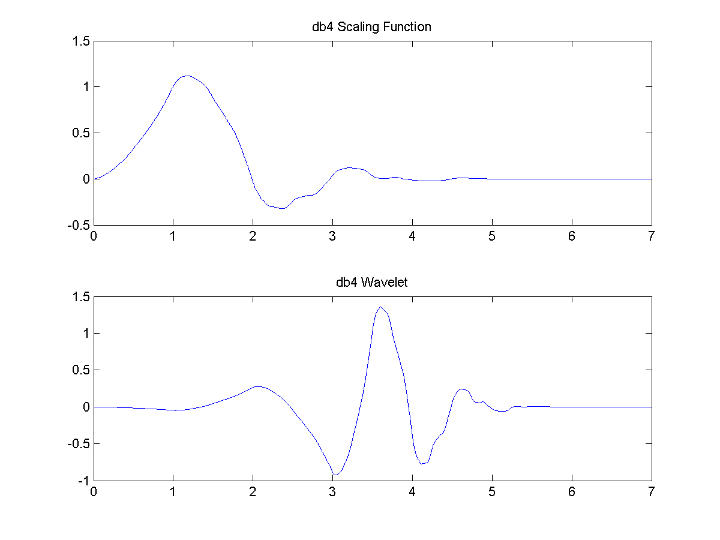In discrete wavelet analysis, the analysis and synthesis filters are of more interest than the associated scaling function and wavelet. You can use `wfilters` to obtain the analysis and synthesis filters.

Obtain the decomposition (analysis) and reconstruction (synthesis) filters for the B-spline biorthogonal wavelet. Specify 3 vanishing moments in the synthesis wavelet and 5 vanishing moments in the analysis wavelet. Plot the filters’ impulse responses.

```[LoD,HiD,LoR,HiR] = wfilters('bior3.5'); subplot(221); stem(LoD); title('Lowpass Analysis Filter'); subplot(222); stem(HiD); title('Highpass Analysis Filter'); subplot(223); stem(LoR); title('Lowpass Synthesis Filter'); subplot(224); stem(HiR); title('Highpass Synthesis Filter');```### Daubechies Wavelets: dbN

The `dbN` wavelets are the Daubechies’ extremal phase wavelets. `N` refers to the number of vanishing moments. These filters are also referred to in the literature by the number of filter taps, which is 2`N`. More about this family can be found in [Dau92] page 195. Enter `waveinfo('db')` at the MATLAB® command prompt to obtain a survey of the main properties of this family.

Daubechies Wavelets db4 on the Left and db8 on the Right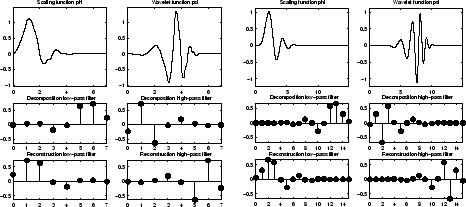The `db1` wavelet is also known as the Haar wavelet. The Haar wavelet is the only orthogonal wavelet with linear phase. Using `waveinfo('haar')`, you can obtain a survey of the main properties of this wavelet.

### Symlet Wavelets: symN

The `symN` wavelets are also known as Daubechies’ least-asymmetric wavelets. The symlets are more symmetric than the extremal phase wavelets. In `symN`, N is the number of vanishing moments. These filters are also referred to in the literature by the number of filter taps, which is 2`N`. More about symlets can be found in [Dau92], pages 198, 254-257. Enter `waveinfo('sym')` at the MATLAB command prompt to obtain a survey of the main properties of this family.

Symlets sym4 on the Left and sym8 on the Right### Coiflet Wavelets: coifN

Coiflet scaling functions also exhibit vanishing moments. In `coifN`, `N` is the number of vanishing moments for both the wavelet and scaling functions. These filters are also referred to in the literature by the number of filter coefficients, which is 3`N`. For the coiflet construction, see [Dau92] pages 258–259. Enter `waveinfo('coif')` at the MATLAB command prompt to obtain a survey of the main properties of this family.

Coiflets coif3 on the Left and coif5 on the Right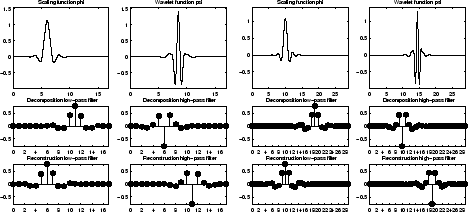If s is a sufficiently regular continuous time signal, for large j the coefficient $〈s,{\varphi }_{-j,k}〉$ is approximated by ${2}^{-j/2}s\left({2}^{-j}k\right)$.

If s is a polynomial of degree d, dN – 1, the approximation becomes an equality. This property is used, connected with sampling problems, when calculating the difference between an expansion over the φj,l of a given signal and its sampled version.

### Biorthogonal Wavelet Pairs: biorNr.Nd

While the Haar wavelet is the only orthogonal wavelet with linear phase, you can design biorthogonal wavelets with linear phase.

Biorthogonal wavelets feature a pair of scaling functions and associated scaling filters — one for analysis and one for synthesis.

There is also a pair of wavelets and associated wavelet filters — one for analysis and one for synthesis.

The analysis and synthesis wavelets can have different numbers of vanishing moments and regularity properties. You can use the wavelet with the greater number of vanishing moments for analysis resulting in a sparse representation, while you use the smoother wavelet for reconstruction.

See [Dau92] pages 259, 262–285 and [Coh92] for more details on the construction of biorthogonal wavelet bases. Enter `waveinfo('bior')` at the command line to obtain a survey of the main properties of this family.

The following code returns the B-spline biorthogonal reconstruction and decomposition filters with 3 and 5 vanishing moments and plots the impulse responses.

The impulse responses of the lowpass filters are symmetric with respect to the midpoint. The impulse responses of the highpass filters are antisymmetric with respect to the midpoint.

```[LoD,HiD,LoR,HiR] = wfilters('bior3.5'); subplot(221); stem(LoD); title('Lowpass Analysis Filter'); subplot(222); stem(HiD); title('Highpass Analysis Filter'); subplot(223); stem(LoR); title('Lowpass Synthesis Filter'); subplot(224); stem(HiR); title('Highpass Synthesis Filter');```#### Reverse Biorthogonal Wavelet Pairs: rbioNr.Nd

This family is obtained from the biorthogonal wavelet pairs previously described.

You can obtain a survey of the main properties of this family by typing `waveinfo('rbio')` from the MATLAB command line.

Reverse Biorthogonal Wavelet rbio1.5### Meyer Wavelet: meyr

Both ψ and φ are defined in the frequency domain, starting with an auxiliary function ν (see [Dau92] pages 117, 119, 137, 152). By typing `waveinfo('meyr')` at the MATLAB command prompt, you can obtain a survey of the main properties of this wavelet.

Meyer Wavelet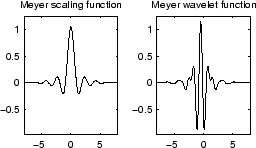The Meyer wavelet and scaling function are defined in the frequency domain:

• Wavelet function

and

where

• Scaling function

By changing the auxiliary function, you get a family of different wavelets. For the required properties of the auxiliary function ν (seeReferences for more information). This wavelet ensures orthogonal analysis.

The function ψ does not have finite support, but ψ decreases to 0 when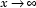, faster than any inverse polynomial

$\forall n\in Ν,\exists {C}_{n}$ such that $|\psi \left(x\right)|\le {C}_{n}{\left(1+{|x|}^{2}\right)}^{-n}$

This property holds also for the derivatives

$\forall k\in N,\forall n\in N,\exists {C}_{k,n}$, such that $|{\psi }^{\left(k\right)}x|\le {C}_{k,n}\left(1+{|x|}^{2}\right)-n$

The wavelet is infinitely differentiable.

Note

Although the Meyer wavelet is not compactly supported, there exists a good approximation leading to FIR filters that you can use in the DWT. Enter `waveinfo('dmey')` at the MATLAB command prompt to obtain a survey of the main properties of this pseudo-wavelet.

### Gaussian Derivatives Family: gaus

This family is built starting from the Gaussian function $f\left(x\right)={C}_{p}{e}^{-{x}^{2}}$ by taking the pth derivative of  f.

The integer p is the parameter of this family and in the previous formula, Cp is such that ${‖{f}^{\left(p\right)}‖}^{2}=1$ where f (p) is the pth derivative of f.

You can obtain a survey of the main properties of this family by typing `waveinfo('gaus')` from the MATLAB command line.

Gaussian Derivative Wavelet gaus8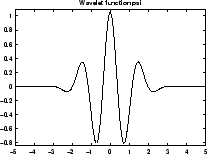### Mexican Hat Wavelet: mexh

This wavelet is proportional to the second derivative function of the Gaussian probability density function. The wavelet is a special case of a larger family of derivative of Gaussian (DOG) wavelets. It is also known as the Ricker wavelet.

There is no scaling function associated with this wavelet.

Enter `waveinfo('mexh')` at the MATLAB command prompt to obtain a survey of the main properties of this wavelet.

You can compute the wavelet with `wavefun`.

```[psi,xval] = wavefun('mexh',10); plot(xval,psi); title('Mexican Hat Wavelet');```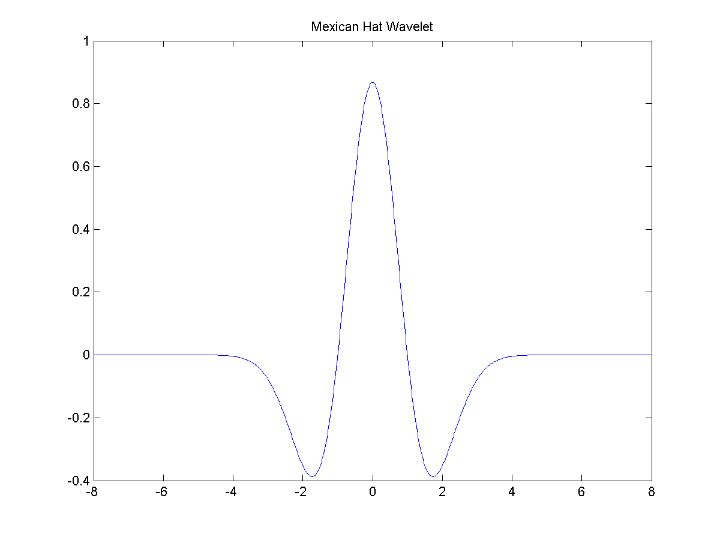### Morlet Wavelet: morl

Both real-valued and complex-valued versions of this wavelet exist. Enter `waveinfo('morl')` at the MATLAB command line to obtain the properties of the real-valued Morlet wavelet.

The real-valued Morlet wavelet is defined as:

`$\psi \left(x\right)=C{e}^{-{x}^{2}}\mathrm{cos}\left(5x\right)$`

The constant C is used for normalization in view of reconstruction.

```[psi,xval] = wavefun('morl',10); plot(xval,psi); title('Real-valued Morlet Wavelet');```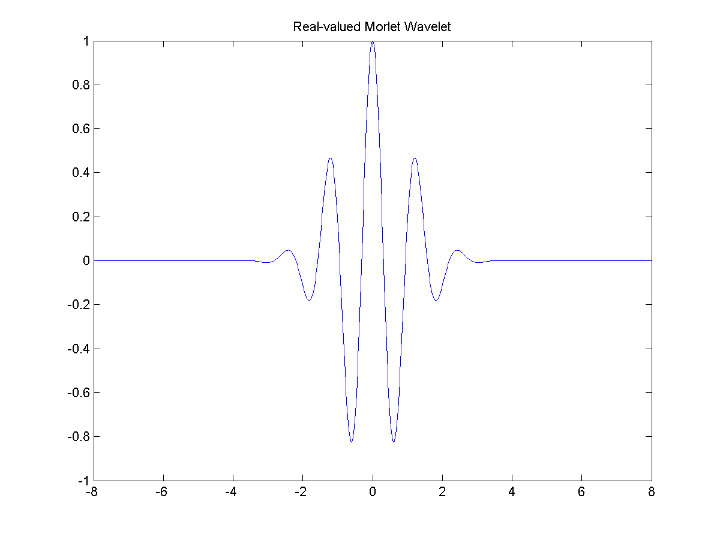The Morlet wavelet does not technically satisfy the admissibility condition..

Some other real wavelets are available in the toolbox.

### Complex Wavelets

The toolbox also provides a number of complex-valued wavelets for continuous wavelet analysis. Complex-valued wavelets provide phase information and are therefore very important in the time-frequency analysis of nonstationary signals.

#### Complex Gaussian Wavelets: cgau

This family is built starting from the complex Gaussian function

$f\left(x\right)={C}_{p}{e}^{-ix}{e}^{-{x}^{2}}$ by taking the pth derivative of f. The integer p is the parameter of this family and in the previous formula, Cp is such that

${‖{f}^{\left(p\right)}‖}^{2}=1$ where f (p) is the pth derivative of f.

You can obtain a survey of the main properties of this family by typing `waveinfo('cgau')` from the MATLAB command line.

Complex Gaussian Wavelet cgau8#### Complex Morlet Wavelets: cmor

See [Teo98] pages 62–65.

A complex Morlet wavelet is defined by

`$\psi \left(x\right)=\frac{1}{\sqrt{\pi {f}_{b}}}{e}^{2i\pi {f}_{c}x}{e}^{\frac{{x}^{2}}{{f}^{b}}}$`

depending on two parameters:

• fb is a bandwidth parameter.

• fc is a wavelet center frequency.

You can obtain a survey of the main properties of this family by typing `waveinfo('cmor')` from the MATLAB command line.

Complex Morlet Wavelet morl 1.5-1#### Complex Frequency B-Spline Wavelets: fbsp

See [Teo98] pages 62–65.

A complex frequency B-spline wavelet is defined by

`$\psi \left(x\right)=\sqrt{{f}_{b}}{\left(\text{sinc}\left(\frac{{f}_{b}x}{m}\right)\right)}^{m}{e}^{2i\pi {f}_{c}x}$`

depending on three parameters:

• m is an integer order parameter (m ≥ 1).

• fb is a bandwidth parameter.

• fc is a wavelet center frequency.

You can obtain a survey of the main properties of this family by typing `waveinfo('fbsp')` from the MATLAB command line.

Complex Frequency B-Spline Wavelet fbsp 2-0.5-1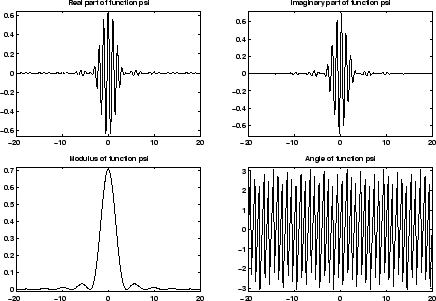#### Complex Shannon Wavelets: shan

See [Teo98] pages 62–65.

This family is obtained from the frequency B-spline wavelets by setting m to 1.

A complex Shannon wavelet is defined by

`$\psi \left(x\right)=\sqrt{{f}_{b}}\text{sinc}\left({f}_{b}x\right){e}^{2i\pi {f}_{c}x}$`

depending on two parameters:

• fb is a bandwidth parameter.

• fc is a wavelet center frequency.

You can obtain a survey of the main properties of this family by typing `waveinfo('shan')` from the MATLAB command line.

Complex Shannon Wavelet shan 0.5-1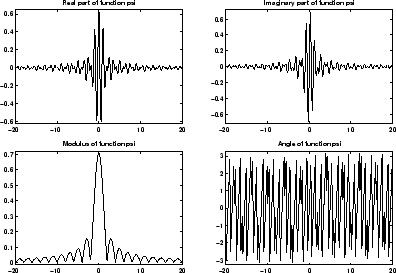## SupportGet trial now